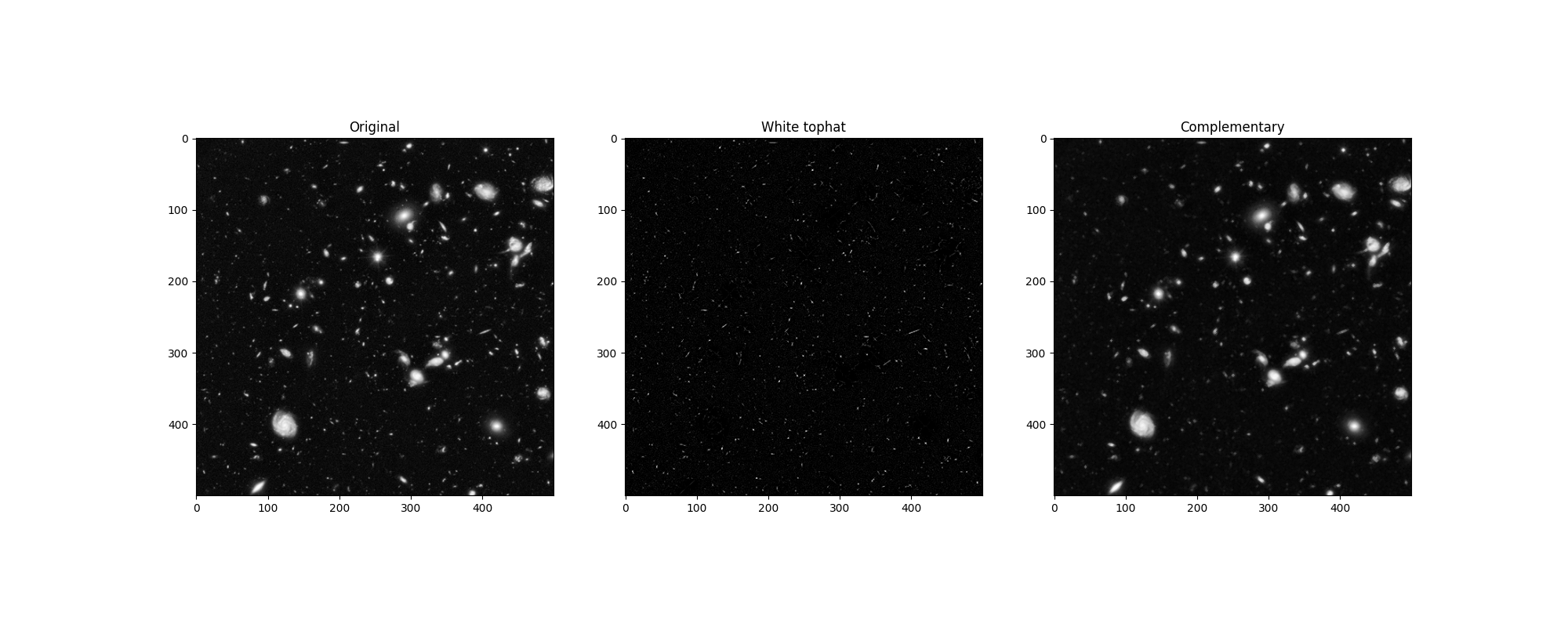# Removing small objects in grayscale images with a top hat filter¶

This example shows how to remove small objects from grayscale images. The top-hat transform 1 is an operation that extracts small elements and details from given images. Here we use a white top-hat transform, which is defined as the difference between the input image and its (mathematical morphology) opening.

1

https://en.wikipedia.org/wiki/Top-hat_transform```import numpy as np
import matplotlib.pyplot as plt

from skimage import data
from skimage import color, morphology

image = color.rgb2gray(data.hubble_deep_field())[:500, :500]

footprint = morphology.disk(1)
res = morphology.white_tophat(image, footprint)

fig, ax = plt.subplots(ncols=3, figsize=(20, 8))
ax.set_title('Original')
ax.imshow(image, cmap='gray')
ax.set_title('White tophat')
ax.imshow(res, cmap='gray')
ax.set_title('Complementary')
ax.imshow(image - res, cmap='gray')

plt.show()
```

Total running time of the script: ( 0 minutes 0.675 seconds)

Gallery generated by Sphinx-Gallery